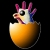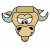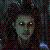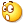Python vs JavaScript

Raymin 发布于 2012/03/19 18:31

【推荐阅读】滴滴出行的数据架构和信息流处理思路解析->>>Python 是个比较成熟的语言，运行速度在几年前是快于 JavaScript 的。但这些年
JavaScript 的解释器发展很快，特别是 Google 的 V8 和 Mozilla 的 SpiderMonkey，

测试环境：
CPU：            Intel(R) Pentium(R) CPU G620 @ 2.60GHz 双核

Google JS 引擎： 来自 Node.js v0.6.12 (命令：node)
Mozilla JS 引擎：来自 xulrunner-11.0 (命令：xpcshell)
Python：         Debian 自带的 Python 2.6.6 (命令：python)
PyPy：           pypy-1.8 (命令：pypy)

先测试简单的循环累加，测试代码：

testSum.js

var i, j, s;

for (i = 0; i < 100; i++) {
s = 0;
for (j = 0; j < 100000; j++)
s += j;
}

if ('function' == typeof(print))
print(i, s);
else
console.log(i, s);

testSum.py

i = 0
while i < 100:
s = 0
j = 0
while j < 100000:
s += j
j += 1
i += 1

print i, s

测试结果：
time node testSum.js     : 0m0.052s
time xpcshell testSum.js : 0m1.808s
time python testSum.py   : 0m2.199s
time pypy testSum.py     : 0m0.188s

再测试 Dict 操作，测试代码：
testDict.js

var D = {};

var i, s, k;

for (i = 0; i < 100000; i++)
D['k' + i] = i;

for (i = 0; i < 100; i++) {
s = 0;
for (k in D)
s += D[k];
}

if ('function' == typeof(print))
print(i, s);
else
console.log(i, s);

testDict.py

D = {}

i = 0
while i < 100000:
D['k%d' % i] = i
i += 1

i = 0
while i < 100:
s = 0
for k in D:
s += D[k]
i += 1

print i, s

测试结果：
time node testDict.js     : 0m3.645s
time xpcshell testDict.js : 0m2.894s
time python testDict.py   : 0m2.954s
time pypy testDict.py     : 0m1.044s

测试总结：

Dict 操作：   pypy < xpcshell < python < node 最慢的是 Node.js (Google V8 引擎)

经过简单的测试，个人感觉 Node.js 在运算速度方面是最快的，但内置的数据结构不

，本人非常看好 PyPy，随着 PyPy 的不断成熟，在大规模的业务逻辑开发中，用 Python

一家之言，欢迎讨论！

以下是话题补充：

@Raymin：补充了 List 操作，参见博文： http://my.oschina.net/raymin/blog/49765 (2012/03/20 12:24)

00引用来自“mallon”的答案

0多虑了。。。这一种没有啥意义的。。各个方面应用的地方都不同。
00m0.052s   0m0.188s   0m1.808s    0m2.199s

Dict 操作：   pypy     < xpcshell < python    < node
0m1.044s   0m2.894s   0m2.954s    0m3.645s

List 操作：   node     < python   < pypy      < xpcshell

0m5.277s   0m6.710s   0m41.702s   3m10.457s

0https://bugzilla.mozilla.org/show_bug.cgi?id=737339